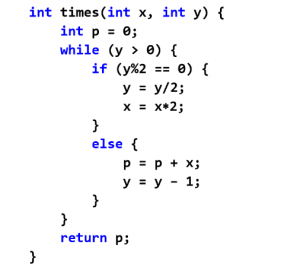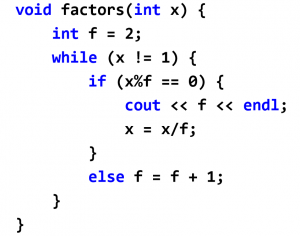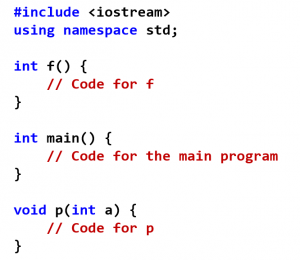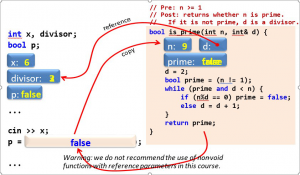You are here: Home > PLC C++ > PLC Chapter VII – Subprogram

## PLC Chapter VII – Subprogram

Programming languages, in particular C++, not only provide a set of basic operations and statements, but also a means to define our own operations and statements. We call the operations and statements that we define functions and procedures, respectively. Procedures and functions (subprograms) may have parameters. These represent the objects from our program that are used in the subprogram.

Functions are defined as follows:

int times (int x, int y) {
// Code
}Procedures are defined similarly, but without delivering any result:

void factors  (int x) {
// Code
}Subprogram definitions may appear before or after the main program.A function can only be used if previously declared. A function can be declared and used before its code is defined.

Once a subprogram has been declared, it can be used. Functions are used as operations within expressions. Procedures are used as statements. Example:

i = times (3, i + 2) + 1; // function …

factors(i);           // procedure …

Appropriate use of subprograms:

1. Increases readability: programs are better structured and easier to understand.
2. Enables the use of abstraction in the program design.
3. Facilitates code reuse.

SUBPROGRAM WITH PARAMETER PASSING

When a subprogram is called, the arguments are passed to the subprogram, so that its code can be executed:

times (3, i + 2) + …
int times(int x, int y) { … }

Each argument must have the same type as its corresponding parameter. In general, any expression can be the argument of a subprogram:

double maximum (double a, double b);

z = maximum (x, y);

r = maximum (3, gcd(s – 4, i) + alpha);

m = maximum (x, maximum (y + 3, 2*Pi*radius));

An object (a variable) is associated with a value and a memory location. In C++, there are two methods for parameter passing:

a. Passing the value (call-by-value). This is denoted by just declaring the type and the name of the parameter.

b. Passing the memory location (call-by-reference). This is denoted by adding the symbol & next to the parameter type.

void p (int x, int& y) { … }

c. Call-by-value makes a copy of the argument at the beginning of the subprogram. It is equivalent to having, a statement that assigns the value of each argument to the corresponding parameter:

times (3, i + 2)

is equivalent to:

int times (int x, int y) { x = 3; y = i + 2; int p = 0; … }

The effect of call-by-reference is that the parameter becomes the same object (variable) as the argument, i.e., the parameter becomes an alias of the argument.

Example: procedure to swap the value of two variables

void exchange (int& x, int& y) {
int z = x;
x = y;
y = z;
}
exchange (a, b);

Is equivalent to having:

void exchange (int& x, int& y) {
int z = a;
a = b;
b = z;
}Tags: , ,